Courses

# Energy Bands and Intrinsic-Extrinsic Semiconductors Class 12 Notes | EduRev

## Class 12 : Energy Bands and Intrinsic-Extrinsic Semiconductors Class 12 Notes | EduRev

The document Energy Bands and Intrinsic-Extrinsic Semiconductors Class 12 Notes | EduRev is a part of the Class 12 Course Physics Class 12.
All you need of Class 12 at this link: Class 12

1.1) Energy bands in solids:

(i) In solids, the group of closely lying energy levels is known as energy band.

(ii) In solids the energy bands are analogous to energy levels in an atom.

(iii) In solids the atoms are arranged very close to each other. In these atoms there are discrete energy levels of electrons. For the formation of crystal these atoms come close together, then due to nucleus-nucleus, electron-electron and electron-nucleus interactions the discrete energy levels of atom distort and consequently each energy level spits into a large number of closely lying energy levels.

(iv) The number of split energy levels is proportional to the number of atoms interacting with each other. If two atoms interact then each energy level splits into two out of which one will be somewhat above and another will be somewhat below the main energy level. In solids the number of atoms is very large (>>1023). Hence each energy level splits into large number of closely lying energy levels. Being very close to each other these energy levels assume the shape of a band.

(v) In an energy band, there are 1023 energy levels with energy difference of 10–23 ev.

(vi) Curve between energy and distance i.e. U-r curve.

(a) When two atoms are interacting: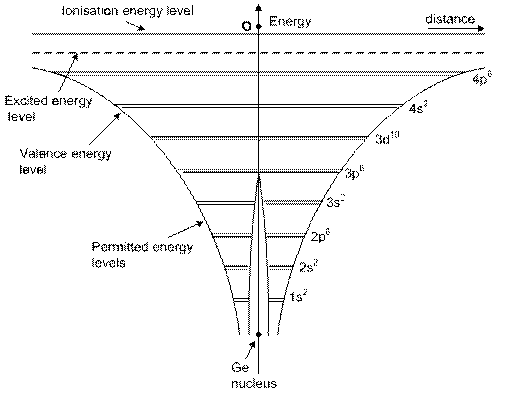(b) When 1023 atoms are mutually interacting: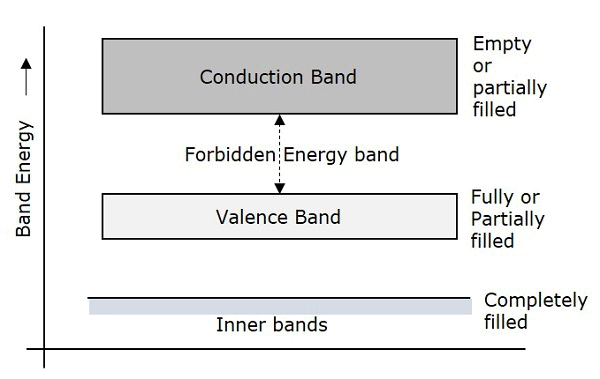2) THREE TYPES OF ENERGY BANDS IN A SOLID, VIZ.

(a) Valence energy band

(b) Conduction energy band

(c) Forbidden energy gap.

• Difference between valence, forbidden and conduction energy bands:
 Valance EnergyBand Forbidden EnergyBand Conduction Energy Band In this band there are valence electrons. No electrons are found in this band In this band the electrons are rarely found This band may be partially or completely filled with electrons. This band is completely empty. This band is either empty or partially filled with electrons. In this band the electrons are not capable of gaining energy from external electric field. In this band the electrons can gain energy from electric field. The electrons in this band do not contribute to electric current. Electrons  in  this bandcontribute in this bandcontribute to electric current. In this band there are electrons of outermost orbit of atom which contribute in band formation, In this band there are electrons which are obtained on breaking the covalent bands, This is the band of maximum energy in which the electrons are  always present. This is the band of minimum energy which is empty. This band can never be empty. This band can be empty.

(1) The conduction band is also known as first permitted energy band or first band.

(2) As there are energy levels f electrons in an atom, similarly there are three specific energy bands for the electrons in the crystal formed by these atoms as shown in the figure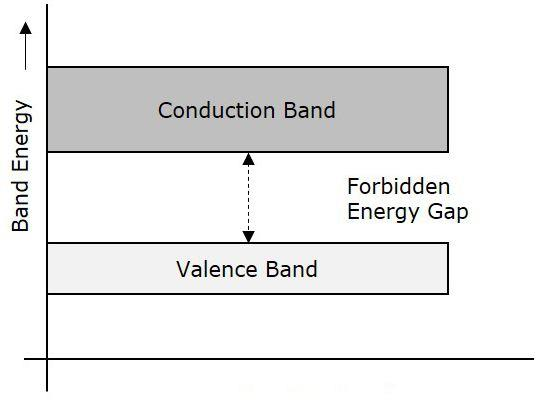Fig: Energy bands

(3) Completely filled energy bands: The energy band, in which maximum possible number of electrons are present according to capacity is known as completely filled bank.

(4) Partially filled energy bands: The energy band, in which number of electrons present is less than the capacity of the band, is known as partially filled energy band.

(5) Electric conduction is possible only in those solids which have empty energy band or partially filled energy band.

(6) Energy gap or Band gap (Eg):

(a) The minimum energy which is necessary for shifting electrons from valence band to conduction band is defined as band gap (Eg)

(b) The forbidden energy gap between the valence band and the conduction band is known as band gap (Eg). i.e. Eg = Ec – Ev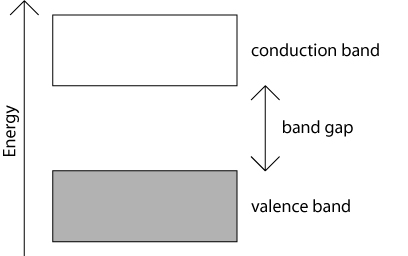(i) On the basis of band structure of crystals, solids are divided in three categories.

(a) Insulators

(b) Semi-conductors

(c) Conductors.

(ii) Difference between Conductors, Semi-conductors and Insulators:

 S.No. Property Conductors Semi­ -conductors Insulators 1. Electrical conductivity and its value Very high10-7 Ω/m Between those of conductors and insulators i.e. 10-7 Ω/m to 10-13 Ω/m Negligible 10-13 Ω/m 2. Resistivity and its value Negligible Less than 10-5Ω-m Between those of conductors and insulators i.e. 10-5Ω-m to 105Ω-m Very high more than 105Ω-m 3. Bandstructure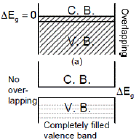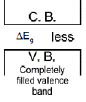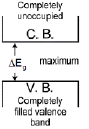4. Energy gap and its value Zero or very small More than that in con­ductors but less than that in insu­lators e.g. in Ge, ΔEg =0.72 eV isSi, ΔEg =1.1 eV in Ga As ΔEg =1.3 eV Very large e.g. in diamond ΔEg = 7 eV 5. Current carriers and current flow Due to free electrons and very high Due to free electrons and holes more than that in insulators Due to free electrons but negligible. 6. Number ofcurrentcarriers(electrons orholes) atordinarytemperature Very high very low negligible
 7, Condition of valence band andconduction band at ordinary temperature The valence and conduction bands are completely filled or conduction band is some what empty (e.g. in Na) Valence band In somewhat empty and conduction band issomewhat filled Valence band is completely filled and conduction band is completely empty. 8. Behaviour at0 K Behaves like a superconductor. Behaves like an insulator Behaves like an insulator 9. Temperature coefficient of resistance (α) Positive Negative Negative 10. Effects oftemperatureonconductivity Conductivity decreases Conductivityincreases Conductivityincreases 11. Onincreasing temperature the number of current carriers Decreases Increases Increases 12. On mixing impurities theirresistance Increases Decreases Remainsunchanged 13. Current flow in these takes place Easily Very slow Does not take place 14. Examples Cu. Ag, Au, Na, Pt, Hg etc. Ge, Si, Ga, As etc. Wood, plastic, mica, diamond, glass etc.

(a)  Semi conducting elements are tetra-valent i.e. there are four electrons in their outermost orbit.
(b)  Their lattice is face centered cubic (F.C.C.)
(c)  The number of electrons or cotters is given by: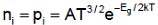i.e. on increasing temperature, the number of current carriers increases.
(d) There are uncharged.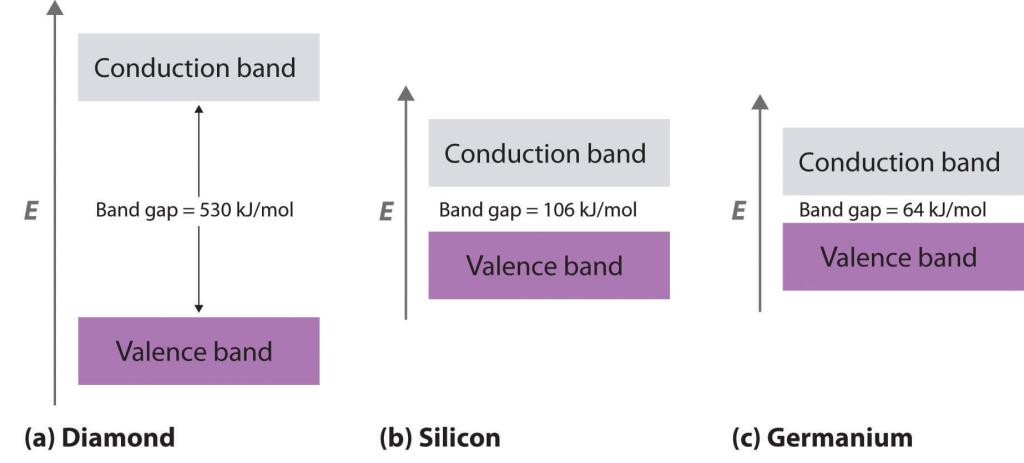Fig: Example of different semi-conductors

(e) Holes or cotters:

(1) The deficiency of electrons in covalent band formation in the valence band in defined as hole or cotter,
(2) These are positively charged. The value of positive charge on them is equal to the electron charge.
(3)  Their effective mass is less than that of electrons.
(4)  In an external electric field, holes move in a direction opposite to that of electrons i,e. they move from positive to negative terminal.
(5)  They contribute to current flow.
(6)  Holes are produced when covalent bonds in valence band break.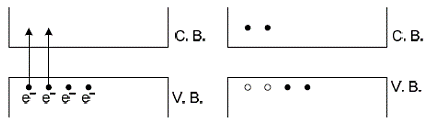Fig: Transfer of electrons from valence band to conduction band

(i) The semiconductors are of two types:

(a) Intrinsic or pure semiconductors

(b) Extrinsic or dopes semiconductors

(ii) Difference between intrinsic and extrinsic semiconductors:

 S.No. Intrinsic semiconductors Extrinsic semiconductors 1. Pure Ge or Si is known as intrinsic semiconductor The semiconductor, resulting from mixing impurity in it, is known as extrinsic semiconductors. 2. Their conductivity is low (because only one electron in 109 contribute) Their conductivity is high 3. The number of free electrons (m in conduction band Is equal to the number of holes pi in valence band.) In these ni ≠pi 4. These are not practically used These are practically used 5. In these the energy gap is very small In these the energy gap is more than that in pure semiconductors. 6. In these the Fermi energy level lies in the middle of valence band and conduction In these the Fermi level shifts towards valence or conduction energy bands.

(a) At absolute zero temperature (0 K) there are no free electrons in them.
(b) At room temperature, the electron-hole pair in sufficient number are produced.
(c)  Electric conduction takes place via both electrons and holes.
(d) The drift velocities of electrons and holes are different.
(e) The drift velocity of electrons (Vdn) is greater than that of holes (Vdp).
(f) The total current is I = In + Jp
(g) In connecting wires the current flows only via electrons.
(h) The current density is given by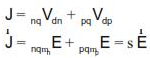Where Vdn = drift velocity of electrons
μn = mobility of electrons
Vdp = drift velocity of holes
μp = mobility of holes

(i) The electric conductivity is given by s = nq(mn + mp)
(j)  Mobility of electron mn = Vdn / E
k) Mobility of holes mp = Vdp/E
(l)  At room temperature sGe > sSi because nGe > nSi
where nGe = 2.5' 1013/cm3 and nSi = 1.4' 1010 /cm3

(a) Doping: The process of mixing impurities of other elements in pure semiconductors is known as doping.

(b) Extrinsic semiconductors: the semiconductors, in which trivalent and pentavalent elements are mixed as impurities, are known as extrinsic semiconductors.

(c) The extrinsic semiconductors are of two types:

(i)  N-type semiconductors

(ii) P-type semiconductors.

Offer running on EduRev: Apply code STAYHOME200 to get INR 200 off on our premium plan EduRev Infinity!

,

,

,

,

,

,

,

,

,

,

,

,

,

,

,

,

,

,

,

,

,

;